##### Geometry For Dummies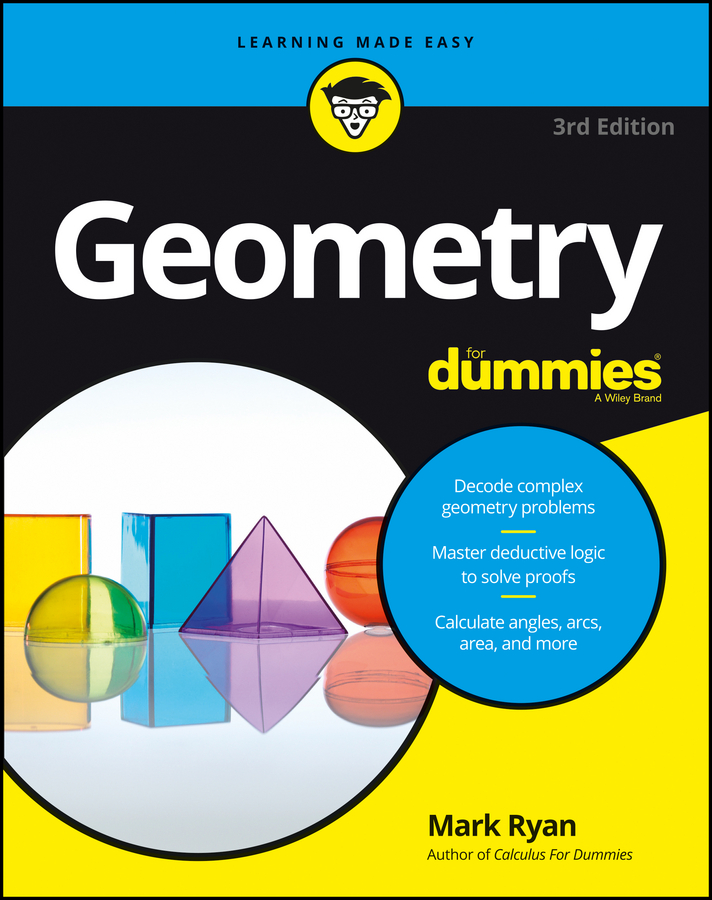There are four addition theorems: two for segments and two for angles. They are used frequently in proofs.

Use the following two addition theorems for proofs involving three segments or three angles:

• Segment addition (three total segments): If a segment is added to two congruent segments, then the sums are congruent.

• Angle addition (three total angles): If an angle is added to two congruent angles, then the sums are congruent.

After you’re comfortable with proofs and know your theorems well, you can abbreviate these theorems as segment addition or angle addition or simply addition; however, when you’re starting out, writing the theorems out in full is a good idea.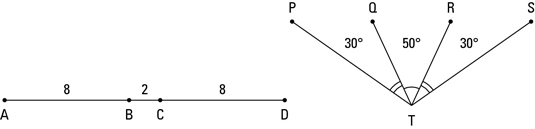The above figure shows you how these two theorems work.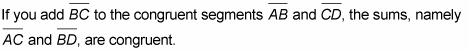In other words, 8 + 2 = 8 + 2. Extraordinary!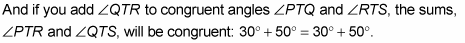Brilliant!

Note: In proofs, you won’t be given segment lengths and angle measures like the ones in the above figure. They are added to the figure so you can more easily see what’s going on.

As you come across different theorems, look carefully at any figures that accompany them. The figures show the logic of the theorems in a visual way that can help you remember the wording of the theorems. Try quizzing yourself by reading a theorem and seeing whether you can draw the figure or by looking at a figure and trying to state the theorem.

• Segment addition (four total segments): If two congruent segments are added to two other congruent segments, then the sums are congruent.

• Angle addition (four total angles): If two congruent angles are added to two other congruent angles, then the sums are congruent.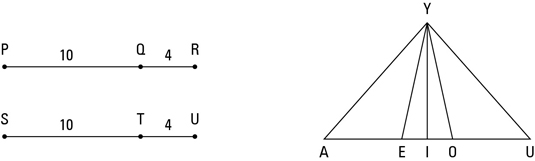Check out the above figure, which illustrates these theorems.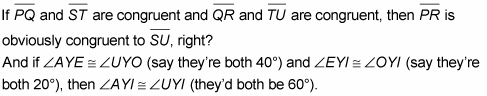Now for a proof that uses segment addition: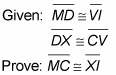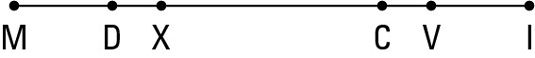You will find what amounts to a game plan for this proof inside the following solution, between the numbered lines.

Statement 1: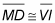Reason for statement 1: Given.

Statement 2: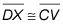Reason for statement 2: Given.

You probably know what comes next: Statement 3 has to use one or both of the givens. To see how you can use the four segments from the givens, make up arbitrary lengths for the segments: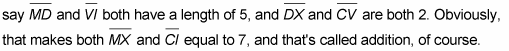So now you’ve got line 3.

Statement 3: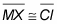Reason for statement 3: If two congruent segments are added to two other congruent segments, then the sums are congruent.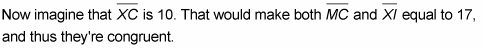This is the three-segment version of segment addition, and that’s a wrap.

Statement 4: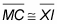Reason for statement 4: If a segment is added to two congruent segments, then the sums are congruent.

By the way, did you see the other way of doing this proof? It uses the three-segment addition theorem in line 3 and the four-segment addition theorem in line 4.

Before looking at the next example, check out these two tips — they’re huge! They can often make a tricky problem much easier and get you unstuck when you’re stuck:

• Use every given. You have to do something with every given in a proof. So if you’re not sure how to do a proof, don’t give up until you’ve asked yourself, “Why did they give me this given?” for every single one of the givens. If you then write down what follows from each given (even if you don’t know how that information will help you), you might see how to proceed. You may have a geometry teacher who likes to throw you the occasional curveball, but in geometry books, the authors generally don’t give you irrelevant givens. And that means that every given is a built-in hint.

• Work backward. Thinking about how a proof will end — what the last and second-to-last lines will look like — is often very helpful. In some proofs, you may be able to work backward from the final statement to the second-to-last statement and then to the third-to-last statement and maybe even to the fourth-to-last. This makes the proof easier to finish because you no longer have to “see” all the way from the given to the prove statement. The proof has, in a sense, been shortened. You can use this process when you get stuck somewhere in the middle of a proof, or sometimes it’s a good thing to try as you begin to tackle a proof.

The following proof shows how you use angle addition: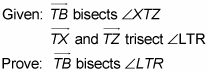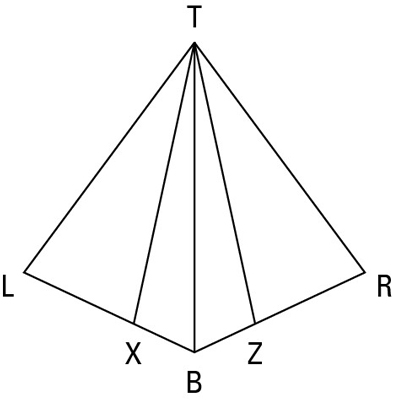This proof includes a partial game plan that deals with the part of the proof where people might get stuck. The only ideas missing from this game plan are the things (which you see in lines 2 and 4) that follow immediately from the two givens.

Statement 1: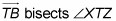Reason for statement 1: Given. (Why would they tell you this? See statement 2.)

Statement 2: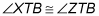Reason for statement 2: If an angle is bisected, then it’s divided into two congruent angles (definition of bisect).

Statement 3: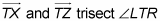Reason for statement 3: Given. (And why would they tell you that?)

Statement 4: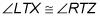Reason for statement 4: If an angle is trisected, then it’s divided into three congruent angles (definition of trisect).

Say you’re stuck here. Try jumping to the end of the proof and working backward. You know that the final statement must be the prove conclusion,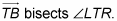Now ask yourself what you’d need to know in order to draw that final conclusion. To conclude that a ray bisects an angle, you need to know that the ray cuts the angle into two equal angles. So the second-to-last statement must be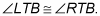And how do you deduce that? Well, with angle addition. The congruent angles from statements 2 and 4 add up to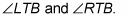That does it.

Statement 5: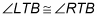Reason for statement 5: If two congruent angles are added to two other congruent angles, then the sums are congruent.

Statement 6: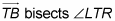Reason for statement 6: If a ray divides an angle into two congruent angles, then it bisects the angle (definition of bisect).